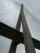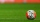Bar

Half of the bar is green, the third is yellow and the remaining 40cm is red. How long is a bar?

Result

x =  240 cm

Solution:

x/2 + x/3 + 40 = x

x = 240

x = 240

Calculated by our simple equation calculator.

Leave us a comment of this math problem and its solution (i.e. if it is still somewhat unclear...):Be the first to comment!To solve this verbal math problem are needed these knowledge from mathematics:

Need help calculate sum, simplify or multiply fractions? Try our fraction calculator. Do you have a linear equation or system of equations and looking for its solution? Or do you have quadratic equation? Do you want to convert length units?

Next similar math problems:

1. Athletic raceBefore a race, you start 4 5/8 feet behind your friend. At the halfway point, you are 3 2/3 feet ahead of your friend. What is the change in distance between you and your friend from the beginning of the race?
2. Unknown numberI think the number. I'll reduce it to its one-third. The result is then increased by one-third, and I get the number 12.
3. NormThree workers planted 3555 seedlings of tomatoes in one dey. First worked at the standard norm, the second planted 120 seedlings more and the third 135 seedlings more than the first worker. How many seedlings were standard norm?
4. NumberI think the number. If I add to its third seven I get same as when to its quarter add 8. Which is the number?
5. Bridge piersOne quarter of the bridge pier is sunk into the ground. Two thirds are in the water. Protruding above the water is 1.20 m long. Determine the height of bridge piers.
6. Unknown number 11That number increased by three equals three times itself?
7. Equation 29Solve next equation: 2 ( 2x + 3 ) = 8 ( 1 - x) -5 ( x -2 )
8. Simple equation 5Solve equation with fractions: X × 3/8 = 1/2
9. TreesFrom the total number of trees in the orchard, there are two-fifths pearls and apples are three eighty. The rest of the trees are 9 ceremonial. How many trees are in the set?
10. Fraction + eqSolve following simple equation with fractions: -5/6(8+5b) = 75 + 5/3b
11. Find unknownFind unknown numerator: 4/8 + _/8 = 1
12. Simple equationSolve for x: 3(x + 2) = x - 18
13. EqnSolve equation with fractions: 2x/3-50=40+x/4
14. PearsThere were pears in the basket, I took two-fifths of them, and left six in the basket. How many pears did I take?
15. Jose and KaitlynJose and Kaitlyn have a contest to see who can throw a baseball the farthest. Kaitlyn wins, with a throw of 200 ft. If Jose threw the ball 3/4 as far as Kaitlyn, how far did Jose throw the ball?
16. Fraction to decimalWrite the fraction 3/22 as a decimal.
17. ZdeněkZdeněk picked up 15 l of water from a 100-liter full-water barrel. Write a fraction of what part of Zdeněk's water he picked.